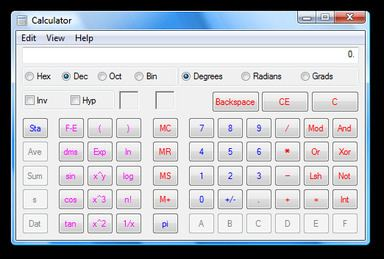# Calculator (Windows)

Updated on
Covid-19
 Type  Software calculatorIncluded with  All versions of Microsoft Windows

Windows Calculator is a software calculator included in all versions of Windows.

## History

A simple arithmetic calculator was first included with Windows 1.0.

In Windows 3.0, a scientific mode was added, which included exponents and roots, logarithms, factorial-based functions, trigonometry (supports radian, degree and gradians angles), base conversions (2, 8, 10, 16), logic operations, statistical functions such as single variable statistics and linear regression.

In Windows 98 and later, it uses an arbitrary-precision arithmetic library, replacing the standard IEEE floating point library. It offers bignum precision for basic operations (addition, subtraction, multiplication, division) and 32 digits of precision for advanced operations (square root, transcendental functions). The largest value that can be represented on the Windows Calculator is currently <1010,000 and the smallest is 10−9,999.

## Windows 7

In Windows 7, separate programmer, statistics, unit conversion, date calculation and worksheets modes were added. Tooltips were removed. Furthermore, Calculator's interface was revamped for the first time since its introduction. The base conversion functions were moved to the programmer mode and statistics functions were moved to the statistics mode. Switching between modes does not preserve the current number, clearing it to 0.

In every mode except programmer mode, one can see the history of calculations. The app was redesigned to accommodate multi-touch. Standard mode behaves as a simple checkbook calculator; entering the sequence 6 * 4 + 12 / 4 - 4 * 5 gives the answer 25. In scientific mode, order of operations is followed while doing calculations (multiplication and division are done before addition and subtraction), which means 6 * 4 + 12 / 4 - 4 * 5 = 7.

In programmer mode, inputting a number in decimal has a lower and upper limit, depending on the data type, and must always be an integer. Data type of number in decimal mode is signed n-bit integer when converting from number in hexadecimal, octal, or binary mode.

On the right of the main Calculator, one can add a panel with date calculation, unit conversion and worksheets. Worksheets allow one to calculate a result of a chosen field based on the values of other fields. Pre-defined templates include calculating a car's fuel economy (mpg and L/100 km), a vehicle lease, and a mortgage. In pre-beta versions of Windows 7, Calculator also provided a Wages template.

## Windows 8.1

While the traditional Calculator is still included with Windows 8.1, a Metro-style Calculator is also present, featuring a full-screen interface as well as normal, scientific, and conversion modes.

## Windows 10

The Calculator in Windows 10 is a universal Windows app. It restores Windows 7 features such as a unit conversions for volume, length, weight, temperature, energy, area, speed, time, power, data, pressure and angle, and the history list which the user can clear.

The traditional Calculator is still included with Windows 10 LTSB, and it was renamed to win32calc.exe. While the original calc.exe file is still present for legacy purposes, it is now just a stub that launches win32calc.exe.

## Features

By default, Calculator runs in standard mode, which resembles a four-function calculator. More advanced functions are available in scientific mode, including logarithms, numerical base conversions, some logical operators, operator precedence, radian, degree and gradians support as well as simple single-variable statistical functions. It does not provide support for user-defined functions, complex numbers, storage variables for intermediate results (other than the classic accumulator memory of pocket calculators), automated polar-cartesian coordinates conversion, or support for two-variables statistics.

Calculator supports keyboard shortcuts; all Calculator features have an associated keyboard shortcut.

Calculator in hexadecimal mode cannot accept or display a hexadecimal number larger than 16 hex digits. The largest number it can handle is therefore 0xFFFFFFFFFFFFFFFF (decimal 18,446,744,073,709,551,615). Any calculations in hex mode which exceed this limit will display a result of zero, even if those calculations would succeed in other modes. In particular, scientific notation is not available in this mode.

## Calculator Plus

Calculator Plus is a separate application for Windows XP and Windows Server 2003 users that adds a 'Conversion' mode over the Windows XP version of the Calculator. The 'Conversion' mode supports unit conversion and currency conversion. Currency exchange rates can be updated using the built-in update feature, which downloads exchange rates from the European Central Bank.

Topics